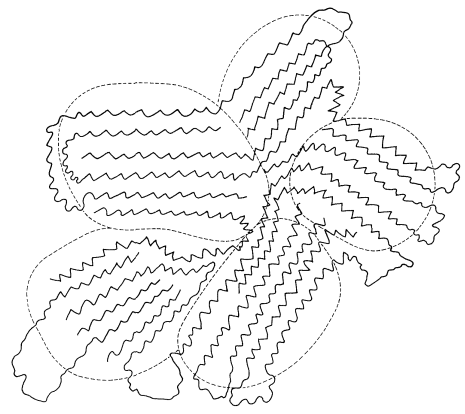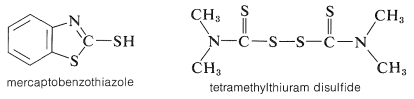# 29.4: Forces Between Polymer Chains

$$\newcommand{\vecs}{\overset { \rightharpoonup} {\mathbf{#1}} }$$ $$\newcommand{\vecd}{\overset{-\!-\!\rightharpoonup}{\vphantom{a}\smash {#1}}}$$$$\newcommand{\id}{\mathrm{id}}$$ $$\newcommand{\Span}{\mathrm{span}}$$ $$\newcommand{\kernel}{\mathrm{null}\,}$$ $$\newcommand{\range}{\mathrm{range}\,}$$ $$\newcommand{\RealPart}{\mathrm{Re}}$$ $$\newcommand{\ImaginaryPart}{\mathrm{Im}}$$ $$\newcommand{\Argument}{\mathrm{Arg}}$$ $$\newcommand{\norm}{\| #1 \|}$$ $$\newcommand{\inner}{\langle #1, #2 \rangle}$$ $$\newcommand{\Span}{\mathrm{span}}$$ $$\newcommand{\id}{\mathrm{id}}$$ $$\newcommand{\Span}{\mathrm{span}}$$ $$\newcommand{\kernel}{\mathrm{null}\,}$$ $$\newcommand{\range}{\mathrm{range}\,}$$ $$\newcommand{\RealPart}{\mathrm{Re}}$$ $$\newcommand{\ImaginaryPart}{\mathrm{Im}}$$ $$\newcommand{\Argument}{\mathrm{Arg}}$$ $$\newcommand{\norm}{\| #1 \|}$$ $$\newcommand{\inner}{\langle #1, #2 \rangle}$$ $$\newcommand{\Span}{\mathrm{span}}$$$$\newcommand{\AA}{\unicode[.8,0]{x212B}}$$

Polymers are produced on an industrial scale primarily, although not exclusively, for use as structural materials. Their physical properties are particularly important in determining their usefulness, be it as rubber tires, sidings for buildings, or solid rocket fuels.Figure 29-3: Representation of attractive interactions between the hydrogens in a crystallite of polyethene. This drawing is incomplete in that it does not show the interactions of the depicted chains with the other chains in front and behind.

Polymers that are not highly cross-linked have properties that depend greatly on the forces that act between the chains. By way of example, consider a polymer such as polyethene which, in a normal commercial sample, will be made up of molecules having 1000 to 2000 $$\ce{CH_2}$$ groups in continuous chains. Because the material is a mixture of different molecules, it is not expected to crystallize in a conventional way.$$^2$$ Nonetheless, x-ray diffraction shows polyethene to have very considerable crystalline character, there being regions as large as several hundred angstrom units in length, which have ordered chains of $$\ce{CH_2}$$ groups oriented with respect to one another like the chains in crystalline low-molecular-weight hydrocarbons. These crystalline regions are called crystallites (Figure 29-3). Between the crystallites of polyethene are amorphous, noncrystalline regions in which the polymer chains are essentially randomly ordered with respect to one another (Figure 29-4). These regions constituted crystal defects.Figure 29-4: Schematic diagram of crystallites (enclosed by dashed lines) in a largely crystalline polymer.

The forces between the chains in the crystallites of polyethene are the so-called van der Waals or dispersion forces, which are the same forces acting between hydrocarbon molecules in the liquid and solid states, and, to a lesser extent, in the vapor state. These forces are relatively weak and arise through synchronization of the motions of the electrons in the separate atoms as they approach one another. The attractive force that results is rapidly overcome by repulsive forces when the atoms get very close to one another (see Figure 12-9, which shows how the potential energy between a pair of atoms varies with the internuclear distance). The attractive intermolecular forces between pairs of hydrogens in the crystallites of polyethene are only about $$0.1$$-$$0.2 \: \text{kcal mol}^{-1}$$ per pair, but for a crystalline segment of 1000 $$\ce{CH_2}$$ units, the sum of these interactions could well be greater than the $$\ce{C-C}$$ bond strengths. Thus when a sample of the crystalline polymer is stressed to the point at which it fractures, carbon-carbon bonds are broken and radicals that can be detected by esr spectroscopy (Section 27-9) are generated.

In other kinds of polymers, even stronger intermolecular forces can be produced by hydrogen bonding. This is especially important in the polyamides, such as the nylons, of which nylon 66 is most widely used (Figure 29-5).Figure 29-5: Possible hydrogen-bonded structure for crystallites of nylon 66, an amide-type polymer of hexanedioic acid and 1,6-hexanediamine.

The effect of temperature on the physical properties of polymers is very important to their practical uses. At low temperatures, polymers become hard and glasslike because the motions of the segments of the polymer chains with relation to each other are slow. The approximate temperature below which glasslike behavior is apparent is called the glass temperature and is symbolized by $$T_g$$. When a polymer containing crystallites is heated, the crystallites ultimately melt, and this temperature is usually called the melting temperature and is symbolized as $$T_m$$. Usually, the molding temperature will be above $$T_m$$ and the mechanical strength of the polymer will diminish rapidly as the temperature approaches $$T_m$$.

Another temperature of great importance in the practical use of polymers is the temperature at which thermal breakdown of the polymer chains occurs. Decomposition temperatures obviously will be sensitive to impurities, such as oxygen, and will be influenced strongly by the presence of inhibitors, antioxidants, and so on. Nonetheless, there will be a temperature (usually rather high, $$200^\text{o}$$ to $$400^\text{o}$$) at which uncatalyzed scission of the bonds in a chain will take place at an appreciable rate and, in general, one cannot expect to prevent this type of reaction from causing degradation of the polymer. Clearly, if this degradation temperature is comparable to $$T_m$$, as it is for polypropenenitrile (polyacrylonitrile), difficulties are to be expected in simple thermal molding of the plastic. This difficulty is overcome in making polypropenenitrile (Orlon) fibers by dissolving the polymer in $$\ce{N}$$,$$\ce{N}$$-dimethylmethanamide and forcing the solution through fine holes into a heated air space where the solvent evaporates.

Physical properties such as tensile strength, x-ray diffraction pattern, resistance to plastic flow, softening point, and elasticity of most polymers can be understood in a general way in terms of crystallites, amorphous regions, the degree of flexibility of the chains, cross-links, and the strength of the forces acting between the chains (dispersion forces, hydrogen bonding, etc.). A good way to appreciate the interaction between the physical properties and structure is to start with a rough classification of properties of solid polymers according to the way the chains are disposed in relation to each other.

1. An amorphous polymer is one with no crystallites. If the attractive forces between the chains are weak and if the motions of the chain are not in some way severely restricted as by cross-linking or large rotational barriers, such a polymer would be expected to have low tensile strength and when stressed undergo plastic flow in which the chains slip by one another.
2. An unoriented crystalline polymer is one which is considerably crystallized but has the crystallites essentially randomly oriented with respect to one another, as in Figure 29-4. When such polymers are heated they often show rather sharp $$T_m$$ points, which correspond to the melting of the crystallites. Above $$T_m$$, these polymers are amorphous and undergo plastic flow, which permits them to be molded. Other things being the same, we expect $$T_m$$ to be higher for polymers with stiff chains (high barriers to internal rotation).
3. An oriented crystalline polymer is one in which the crystallites are oriented with respect to one another, usually as the result of a cold-drawing process. Consider a polymer such as nylon, which has strong intermolecular forces and, when first prepared, is in an unoriented state like the one represented by Figure 29-4. When the material is subjected to strong stress in one direction, usually above $$T_g$$ so that some plastic flow can occur, the material elongates and the crystallites are drawn together and oriented along the direction of the applied stress (Figure 29-6).Figure 29-6: Schematic representation of an oriented crystalline polymer produced by drawing the polymer in the horizontal direction. The crystalline regions are enclosed with dashed lines.

An oriented crystalline polymer usually has a much higher tensile strength than the unoriented polymer. Cold drawing is an important step in the production of synthetic fibers.

1. Elastomers usually are amorphous polymers. The key to elastic behavior is to have highly flexible chains with either sufficiently weak forces between the chains or a sufficiently irregular structure to be unstable in the crystalline state. The tendency for the chains to crystallize often can be considerably reduced by random introduction of methyl groups, which by steric hindrance inhibit ordering of the chains. A useful elastomer needs to have some kind of cross-linked region to prevent plastic flow and flexible enough chains to have a low $$T_g$$. The structure of a polymer of this kind is shown schematically in Figure 29-7; the important difference between this elastomer and the crystalline polymer of Figure 29-4 is the size of the amorphous regions. When tension is applied and the material elongates, the chains in the amorphous regions straighten out and become more nearly parallel. At the elastic limit, a semicrystalline state is reached, which is different from the one produced by cold drawing of a crystalline polymer in that it is stable only while under tension. The forces between the chains are too weak to maintain the crystalline state in the absence of tension. Thus when tension is released, contraction occurs and the original, amorphous polymer is produced. The entropy (Section 4-4B) of the chains is more favorable in the relaxed state than in the stretched state.Figure 29-7: Schematic representation of an elastomer in relaxed and stretched configurations. Many elastomers do not crystallize when elongated.

A good elastomer should not undergo plastic flow in either the stretched or relaxed state, and when stretched should have a "memory" of its relaxed state. These conditions are best achieved with natural rubber (cis-poly-2-methyl-1,3-butadiene, cis-polyisoprene; Section 13-4) by curing (vulcanizing) with sulfur. Natural rubber is tacky and undergoes plastic flow rather readily, but when it is heated with $$1$$-$$8\%$$ by weight of elemental sulfur in the presence of an accelerator, sulfur cross-links are introduced between the chains. These cross-links reduce plastic flow and provide a reference framework for the stretched polymer to return to when it is allowed to relax. Too much sulfur completely destroys the elastic properties and produces hard rubber of the kind used in cases for storage batteries.

The chemistry of the vulcanization of rubber is complex. The reaction of rubber with sulfur is markedly expedited by substances called accelerators, of which those commonly known as mercaptobenzothiazole and tetramethylthiuram disulfide are examples:Clearly, the double bonds in natural rubber are essential to vulcanization because hydrogenated rubber ("hydrorubber") is not vulcanized by sulfur. The degree of unsaturation decreases during vulcanization, although the decrease is much less than one double bond per atom of sulfur introduced. There is evidence that attack occurs both at the double bond and at the adjacent hydrogen (in a manner similar to some halogenations; Section 14-3A) giving cross-links possibly of the following types:The accelerators probably function by acting as sulfur carriers from the elemental sulfur to the sites of the polymer where the cross-links are formed.

$$^2$$Quite good platelike crystals, about $$100 \: \text{Å}$$ thick, have been formed from dilute solutions of polyethene. In these crystals, $$\ce{CH_2}$$ chains in the anti conformation (Section 5-2) run between the large surfaces of the plates. However, the evidence is strong that when the $$\ce{CH_2}$$ chains reach the surface of the crystal they do not neatly fold over and run back down to the other surface. Instead, the parts of a given chain that are in the crystalline segments appear to be connected at the ends of the crystallites by random loops of disordered $$\ce{CH_2}$$ sequences, something like an old-fashioned telephone switchboard.